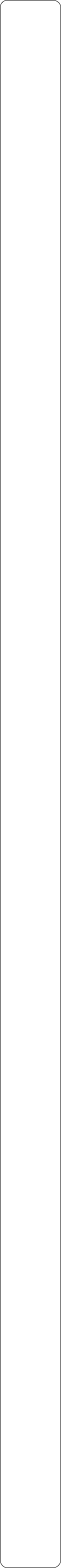Year 5 Objectives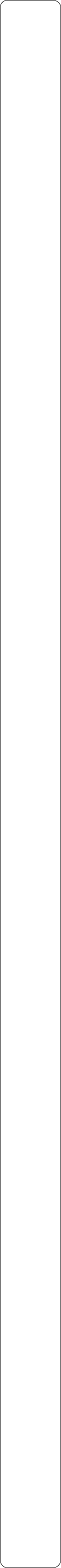Place ValuePre Tasks

(PitStops)

Main TasksPre Tasks

(PitStops)

Main Tasks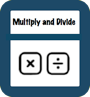Multiplication and Division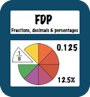Pre Tasks

(PitStops)

Main Tasks

Pre Tasks

(PitStops)

Main Tasks

Number - Fractions- Identify multiples and factors, including finding all factor pairs of a number, and common factors of two numbers

- Know and use the vocabulary of prime numbers, prime factors and composite (non-prime) numbers

- Establish whether a number up to 100 is prime and recall prime numbers up to 19

- Multiply numbers up to 4 digits by a one- or two-digit number using a formal written method, including long multiplication for two-digit numbers

- Multiply/divide numbers mentally drawing upon known facts

- Divide numbers up to 4 digits by a one-digit number using the formal written method of short division and interpret remainders appropriately for the context

- Multiply and divide whole numbers and those involving decimals by 10, 100 and 1000

- Recognise and use square numbers and cube numbers, and the notation for squared (2) and cubed (3)

- Solve problems involving multiplication and division including using their knowledge of factors and multiples, squares and cubes

- Solve problems involving addition, subtraction, multiplication and division and a combination of these, including understanding the meaning of the equals sign- Read, write, order and compare numbers to at least 1 000 000 and determine the value of each digit

- Count forwards or backwards in steps of powers of 10 for any given number up to 1 000 000

- Interpret negative numbers in context, count forwards and backwards with positive and negative whole numbers, including through zero

- Round any number up to 1 000 000 to the nearest 10, 100, 1000, 10 000 and 100 000

- Solve number problems and practical problems that involve all of the above- Read Roman numerals to 1 000 (M) and recognise years written in Roman numerals.

- Add and subtract whole numbers with more than 4 digits

- Add and subtract whole numbers with more than 4 digits, including using formal written methods (columnar addition and subtraction)

- Add and subtract numbers mentally with increasingly large numbers

- Use rounding to check answers to calculations and determine, in the context of a problem, levels of accuracy

- Solve addition and subtraction multi-step problems in contexts, deciding which operations and methods to use and why.

- Solve problems involving multiplication and division, including scaling by simple fractions and problems involving simple rates

Addition and Subtraction1. -Compare and order fractions whose denominators are all multiples of the same number

- Identify, name and write equivalent fractions of a given fraction, represented visually, including tenths and hundredths

- Recognise mixed numbers and improper fractions and convert from one form to the other and write mathematical statements > 1 as a mixed number [eg., ⅖ + ⅘ = 6/5 = 1⅕

- Add and subtract fractions with the same denominator and denominators that are multiples of the same number

- Multiply proper fractions and mixed numbers by whole numbers, supported by materials and diagrams

- Read and write decimal numbers as fractions [for example, 0.71 = 71/100 ]

- Recognise and use thousandths and relate them to tenths, hundredths and decimal equivalents

- Round decimals with two decimal places to the nearest whole number and to one decimal place

- Read, write, order and compare numbers with up to three decimal places

- Solve problems involving number up to three decimal places

- Recognise the % symbol and understand that per cent relates to ‘number of parts per hundred’, and write percentages as a fraction with denominator 100, and as a decimal

- Solve problems which require knowing percentage and decimal equivalents of ½, ¼, ⅕, ⅖, ⅘ and those fractions with a denominator of a multiple of 10 or 25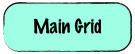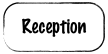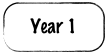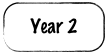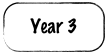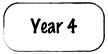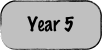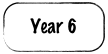Solutions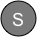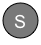StopsGap

StopsGap

StopsGap

StopsGap